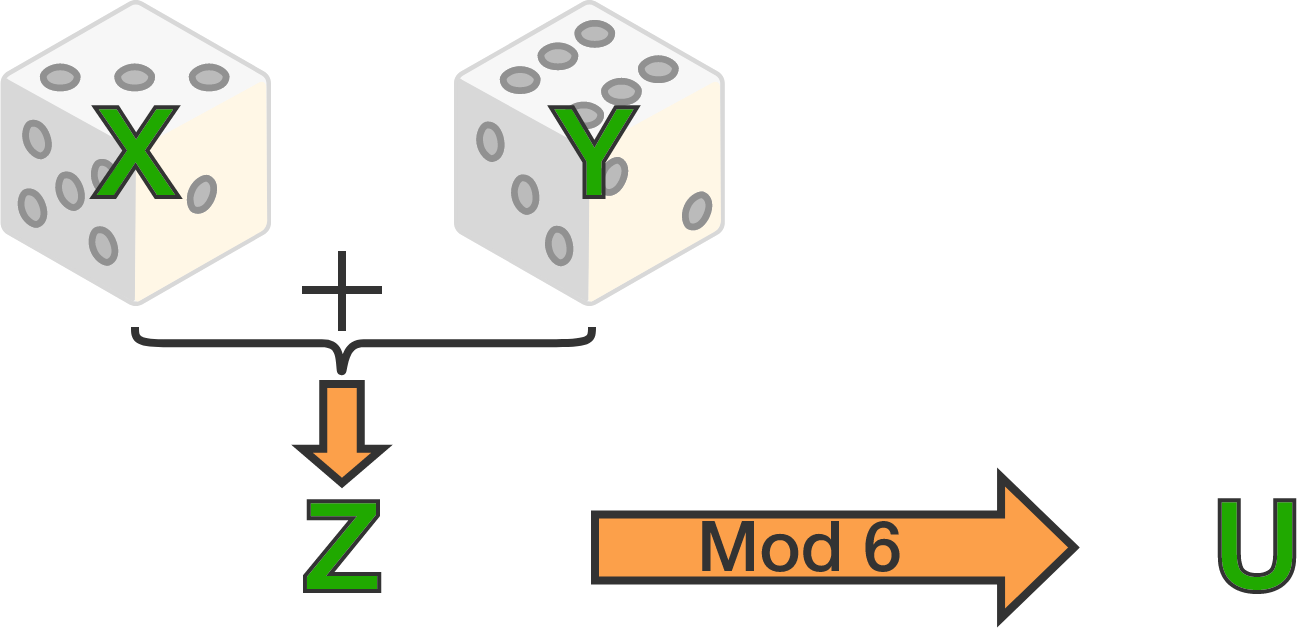# Independence, part 2

Probability Level 3A fair die is thrown two times independently. Let $X$ and $Y$ be the random variable indicating the outcomes of these two throws. Define $Z = X + Y,$ and define $U$ as the remainder when $Z$ is divided by $6$.

Which of the following statements are true?

1. $X$ and $Z$ are independent.
2. $X$ and $U$ are independent.
3. $Z$ and $U$ are independent.
×

Problem Loading...

Note Loading...

Set Loading...